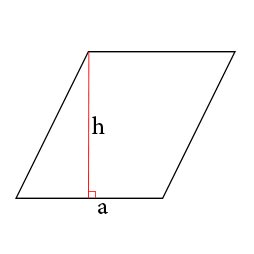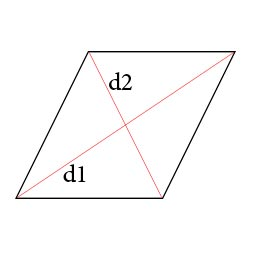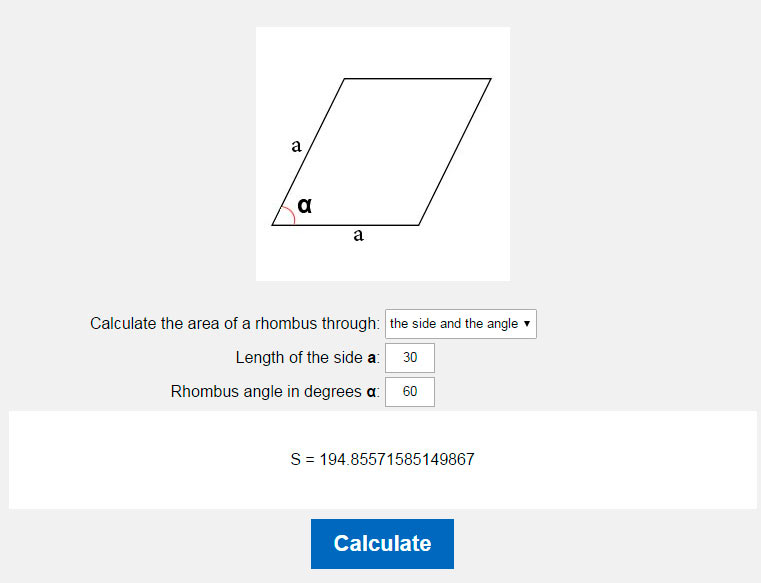# Area of a rhombus calculator. Calculate the area of a rhombus.

You could easy calculate rhombus area by our online calculator.Calculate the area of a rhombus through: base and heightdiagonalthe side and the angle Length of the side a: Height of the rhombus h:

There are several formulas with which you can calculate the area of a rhombus.

1. We can calculate the area of a rhombus knowing the height and length of the base of the following formula:
S=ah
where a - is a side, h - the height of the rhombus2. Just rhombus area can be found across the length and angle of the sides of a rhombus:
S=a2sin(α)
where a - is a party, α - angle in degrees rhombus3. Still, we can learn a rhombus square knowing the length of the two diagonals
S=d1d2/2
where d 1 and d 2 - is the diagonal of the rhombus### Calculate the area of a rhombus through the base and height### Calculate the area of a rhombus through the side and the angle### Calculate the area of a rhombus through a diagonalHOME
RESOURCES